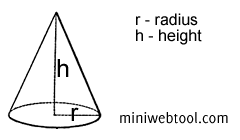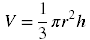×

# Volume of a Cone Calculator## About Volume of a Cone Calculator

The Volume of a Cone Calculator is used to help you find the volume of a cone.

## Volume of a Cone Formula

The following is the calculation formula for the volume of a cone:Where:
V = volume of a cone
π = 3.141592654
h = height

## All of Our Miniwebtools (Sorted by Name):

•{{name}}
{{title}}

Miniwebtool

If you like Volume of a Cone Calculator, please consider adding a link to this tool by copy/paste the following code:

Watch Videos: